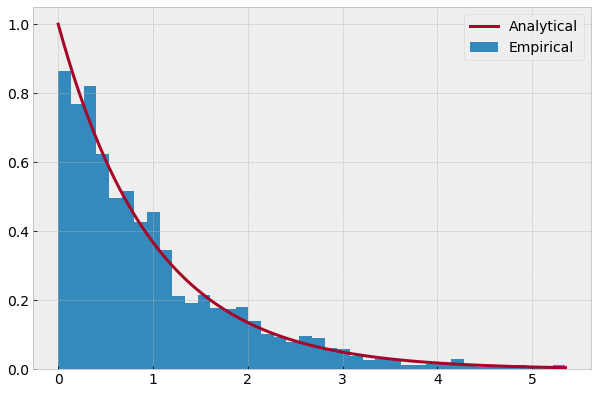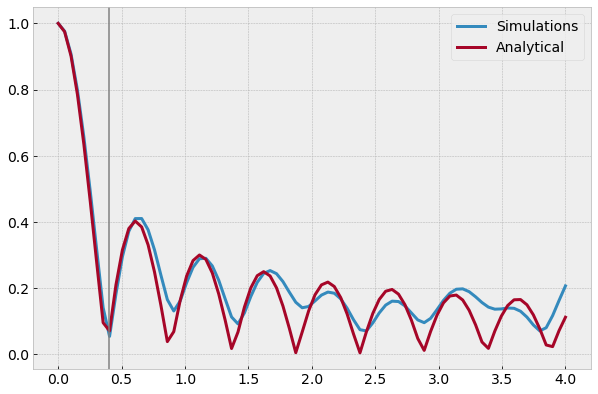I'm about to setup simulations that use a multi-path channel model. Since I wanted to rely on GNU Radio's channel model blocks, I had a look at their implementation and think I found some flaws.

With the fixes, the output looks pretty OK. I used the following iPython notebook to test the statistical properties of the Rayleigh fading block.

In :
%matplotlib inline
import numpy as np
import matplotlib.pyplot as plt
plt.style.use('bmh')
plt.rcParams['figure.figsize']   = (10, 10.0*2/3)
plt.rcParams['font.size']        = 14
plt.rcParams['lines.linewidth']  = '3'

SimulationsÂ¶

I created some channel coefficients by piping 1s through the block.

In :
N = 12              # N sinusoids
v = 75 / 3.6        # speed (km/h to m/s)
f = 6e9             # freq
c = 3e8             # speed of light
f_d = f * v / 3e8   # max. Doppler
samp_rate = 10e6    # 10 MHz
N_samp = 1000000    # samples to generate
fDTs = f * v / c / samp_rate
seed = np.random.randint(10000)

from gnuradio import gr, blocks, channels
sig = blocks.vector_source_c(*N_samp, False)
chan = channels.fading_model(N, fDTs, False, 4.0, seed)
snk = blocks.vector_sink_c()

## do simulations
tb = gr.top_block()
tb.connect(sig, chan)
tb.connect(chan, snk)
tb.run()

channel = snk.data()
coef = np.abs(channel)
power = coef**2

Average PowerÂ¶

Since the samples get normalized, I guess the average power is supposed to be 1.

In :
print "Avg. Power: %.2f" % np.mean(power)
Avg. Power: 0.99

Power DistributionÂ¶

The power of Rayleigh distributed channel coeffients should follow an exponential distribution. Since the power is normalized to 1, $\lambda=1$.

In :
# empirical distribution
plt.hist(power, bins=40, normed=True, label='Empirical');

# anlytical distribution
x = np.linspace(0, np.max(power), num=100)
plt.plot(x, np.exp(-x), label='Analytical')
plt.legend(loc=1);AutocorrelationÂ¶

The autocorrelation of the channel coefficients should follow a bessel function

$$R(\tau) = J_0(2 \pi f_d \tau).$$

A well-known characteristic is that this function has a zero-crossing after about the time it takes to move 0.4 times the wave length.

In :
n_w = 4
w = int(n_w * c / f / v * samp_rate)

def autocorr(x, t=1):
return np.corrcoef(np.array([x[0:len(x)-t], x[t:len(x)]]))

gaps = np.round(np.linspace(0, w, num=80))
gaps = gaps.astype(int)

## empirical
acorr = map(lambda x: np.abs(autocorr(channel, x)[0, 1]), gaps)

## analytical
import scipy.special
bessel = map(lambda x: scipy.special.jv(0, 2 * np.pi * f_d / samp_rate * x), gaps)

plt.plot(gaps/c*f*v/samp_rate, acorr, label='Simulations')
plt.plot(gaps/c*f*v/samp_rate, np.abs(bessel), label='Analytical')
plt.legend(loc=1)
plt.axvline(0.4, linewidth=1.5, color='gray');